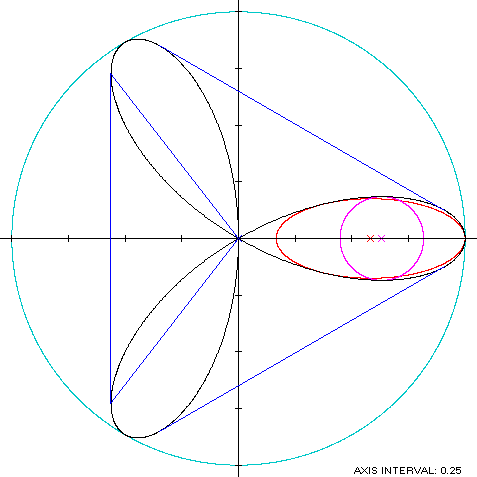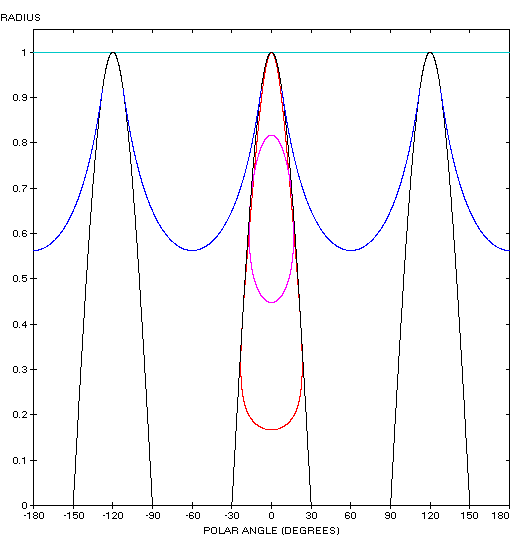Rose
ROSE

Balmoral Software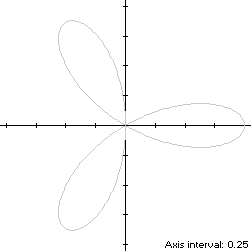A three-lobed rose with a petal on the positive x-axis is a closed curve S with polar equation
r(t) = cos(3t), 0 ≤ t < π
This curve is also known as the trifolium. Movement along S is always counterclockwise, starting at its maximum abscissa point (1,0) and crossing the origin three times. There are two minimum-abscissa points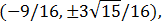the lower of which occurs at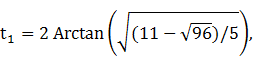so S is non-convex by the multiple local extrema test. The ordinate extrema of S are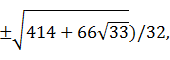so the width x height of its bounding rectangle is### Symmetry

Since
r(t + 2π/3) = cos(3t + 2π) = r(t),
the condition (R2) of Lemma R is satisfied and the rose is rotationally symmetric with period 2π/3.

### Metrics

The perimeter of the rose is 6.682447 and its area is π/4.

### Convex Hull

The convex hull is created by connecting the minimum abscissa points with a vertical line segment of length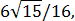and then repeating the pattern by rotating around the origin at 2π/3 intervals. The far end of the lower-left rose petal is reached at t = π/3, so the rounded edge of the petal that occurs for values of t between t1 and π/3 is part of the convex hull, and there are 6 such parts by symmetry. We have
r'(t) = -3sin(3t),
so by (L2), the perimeter of the convex hull is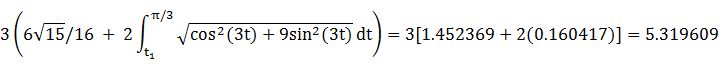which is about 20% shorter than that of the rose.

The line segments of the convex hull create three isosceles triangles with the origin, each having an area of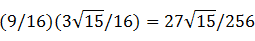as shown in blue in the left diagram below. By (A2), the area of the convex hull is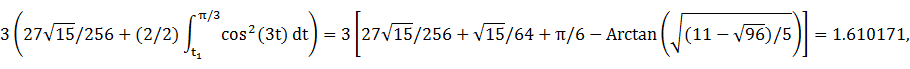which is a little over twice the area of the rose.

### Circumconics

Since the rose is rotationally symmetric with period 2π/3, its circumcircle and circumellipse are the same. The squared-distance function of S
r2(t) = cos2(3t)
is maximized at 1, so that is the circumradius.

### Incircle (lobe)

Consider the x-symmetric right lobe of the rose, where -π/6 ≤ t < π/6. The maximum ordinate point on this lobe is (c,R), where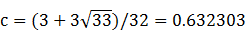and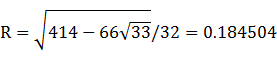The largest circle centered on the x-axis and inscribed in the lobe has radius R and center abscissa c. The circle must be contained within the lobe, so we require that c - R and c + R both be within the abscissa range [0,1] of the lobe, which is true. For verification, we have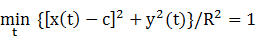### Inellipse (lobe)

Using z = 1 in Lemma E,
d/dt [x(t) - z]y(t) = d/dt [6sin(2t) - 5sin(4t) + sin(8t)]/8
has a zero at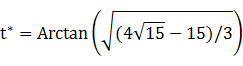The corresponding coordinates are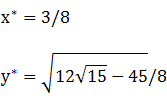We then have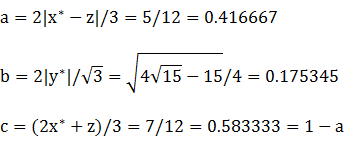For verification, we have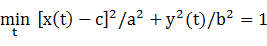### Summary Table

 Perimeter Area Centroid Figure Parameters Incircle (lobe) R =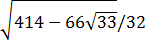1.159273 0.106945 (0.632303,0) Inellipse (lobe) a = 0.416666b = 0.175345 1.937955 0.229526 (0.583334,0) Rose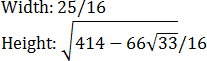6.682447 0.785398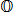Convex hull 5.319609 1.610171 Circumcircle R = 1 6.283185 3.141593